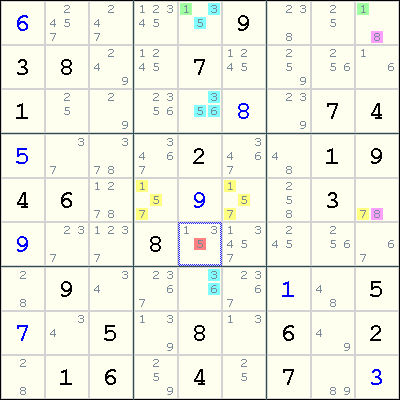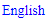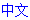# Death Blossom

The Death Blossom technique involves a stem cell of N candidates that sees N petals, each an Almost Locked Set.

It is developed by extending the Aligned Pair Exclusion technique into an arbitrary sized set of non-aligned cells (see Subset Exclusion), with most of the cells belonging to Almost Locked Sets.

### How it worksA Death Blossom consists of a "stem" cell and an Almost Locked Set (or ALS) for each of the stem cell's candidates. The ALS associated with a particular candidate of the stem cell has that number as one of its own candidates, and within the ALS, every cell that has the number as a candidate can see the stem cell. The ALSes can't overlap; i.e., no cell can belong to more than one ALS. Also, there must be at least one number that is a candidate of every ALS, but is not a candidate of the stem cell.

Once we've found a Death Blossom, if an outside cell that doesn't belong to one of the ALSes (and isn't the stem cell) can see every cell in each ALS that has a particular number as a candidate, and the number isn't a candidate of the stem cell, then the number can't be a candidate of the outside cell.

The workings of the Death Blossom is best explained via an example. This example is a scrambled version of the example at Andrew Stuart's Advanced Sudoku Strategies page.

We have a stem cell at r1c9 with two candidates 1 and 8. Observe that r1c9 sees all the 1s in the blue ALS, and r1c9 sees all the 8s in the yellow ALS. These two ALSes are the petals. Now, by a proof by cases:

• If r1c9=1, then the blue ALS gets reduced to a Naked Triple of 3, 5 and 6 and so r6c5<>5.
• If r1c9=8, then r5c9=7, and the remainder of the yellow ALS gets reduced to a Naked Pair of 1 and 5 and so r6c5<>5.

Therefore, we can eliminate 5 from r6c5.

Observe how this is an extension of Aligned Pair Exclusion, whose cells consist of the r6c5, the blue ALS and the yellow ALS. If r6c5=5, then r1c5=1 is forced by the blue ALS, and also r5c9=8 is forced by the yellow ALS, and so r1c9 has no candidates remaining. The Death Blossom technique is derived by working forwards from r1c9.

Note

If the stem cell has exactly two candidates, like the example above, then the same eliminations can be replicated using a ALS-XY-Wing move.

## Sudoku strategies

﻿## Printable Sudoku